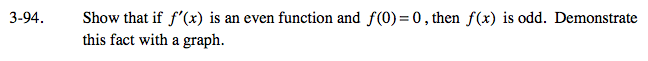### Home > CALC > Chapter 3 > Lesson 3.3.1 > Problem3-94

3-94.

Show that if f ′(x) is an even function and f(0) = 0 , then f(x) is odd. Demonstrate this fact with a graph. Homework Help ✎Sketch different examples of possible f '(x) functions that are both even and go through the origin. Then sketch their antiderivatives f(x).

Make a conjecture about why this will work ONLY if the even derivative goes through the origin? For example: consider even function f '(x) = x² + 1